Courses

FIR Filter Design & Realizations of Digital Filters Notes | Study Signals and Systems - Electrical Engineering (EE)

Electrical Engineering (EE): FIR Filter Design & Realizations of Digital Filters Notes | Study Signals and Systems - Electrical Engineering (EE)

The document FIR Filter Design & Realizations of Digital Filters Notes | Study Signals and Systems - Electrical Engineering (EE) is a part of the Electrical Engineering (EE) Course Signals and Systems.
All you need of Electrical Engineering (EE) at this link: Electrical Engineering (EE)

FIR filter design

In the previous section, digital filters were designed to give a desired frequency response magnitude without regard to the phase response. In many cases a linear phase characteristics is required through the passband of the filter. It can be shown that causal IIR filter cannot produce a linear phase characteristics and only special forms of causal FIR filters can give linear phase.

If {h[n]} represents the impulse response of a discrete time linear system a necessary and sufficient condition for linear phase is that {h[n]} have finite duration , that it be symmetric about its mid point, i.e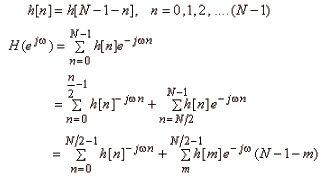For even, we get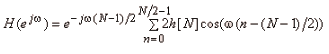For N odd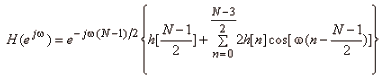For even we get a non-integer delay, which will cause the value of the sequenceto change, [See continuous time implementation of discrete time system, for interpretation of non-integer delay].

One approach to design FIR filters with linear phase is to use windowing.

The easiest way to obtain an FIR filter is to simply truncate the impulse response of an IIR filter. If {hd[n]} is the impulse response of the designed FIR filter, then an FIR filter with impulseresponse {h[n]} can be obtained as follows.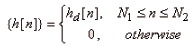This can be thought of as being formed by a product of {hd[n]} and a window function {ω[n]}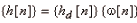where {ω[n]}  is said to be rectangular window and is given byUsing modulation property of Fourier transfer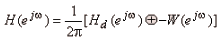For example if  Hd(e) is ideal low pass filter and {ω[n]} is rectangular window is measured version of the ideal low pass frequency response.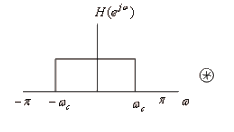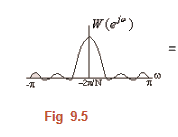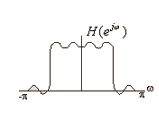In general, the index the main lobe of  W(e), the more H(e) spreading where as the narrower the main lobe (larger N), the closer |H(e)| comes to. In general, we are left with a trade-off of making N large-enough so that smearing is minimized, yet small enough to allow reasonable implementation. Much work has been done on adjusting {ω[n]} to satisfy certain main lobe and side lobe requirements. Some of the commonly used windows are give in below.

(a) Rectangular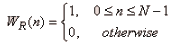(b) Bartlett (or triangle)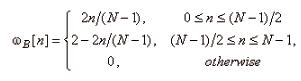(c) Hanning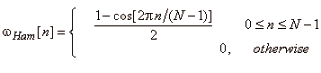(d) Harming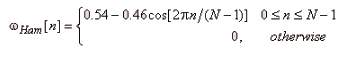(e) Blackman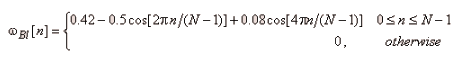(f) Kaiser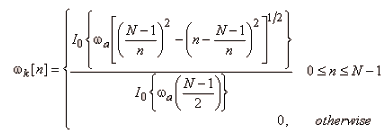where  I0(x) is modified zero-order Bessel function of the first kind given by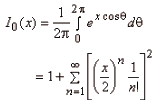The main lobe width and first side lobe attenuation increase as we proceed down the window listed above.

An ideal lowpass filter with linear phase and cut off ωc is characterized by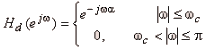The corresponding impulse response is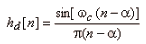Since this is symmetric about n = α , if we change α = (N - 1)/2 and use one of the windows listed above the will get linear phase FIR filter. Transition width and minimum stopped attenuation are listed in the Table 9.3.

 Window Transition Width Minimum stopband attenuation Rectangular 4π/N -21db Bartlett 8π/N -25dB Hanning 8π/N -44dB Hamming 8π/N -53dB Blackman 12π/N -74dB Kaiser variable variable

Table 9.3

We first choose a window that satisfies the minimum attenuation. The transition bandwidth is approximately that allows us to choose the value of N. Actual frequency response characteristic are then calculated and we see if the requirements are met or not. Accordingly N is adjusted parameters for kaiser window are obtained from design formula available for this MATLAB or similar programmes have all there formulas.

Realizations of Digital Filters

We have many realizations of digital filter. Some of these are now discussed. Direct Form Realization - An important class of linear time -invariant systems is characterized by the transfer function.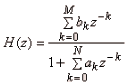A system with input {x[n]} and output {y[n]} could be realized by the following constant coefficient difference equation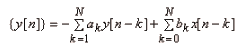A realization of the filter using equation (9.31) is shown in figure (9.6)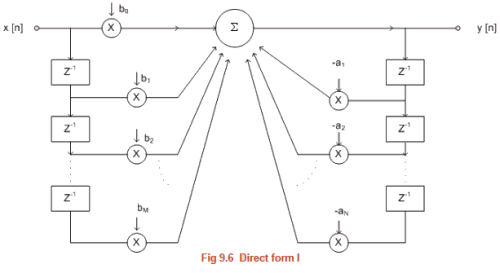The output y[n] is seen to be weighted sum of input x[n] and past inputs x[n - 1],.....x[n - A] and past outputs. Another realization can be obtained by uniting H(z) as product of two transfer functions H1(z) and H2(z), where H1(z) contains only the denominator or poles and H2(z) contains only the numerator or zeros as follows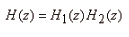where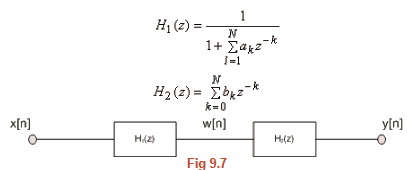The output of the filter is obtained by calculating the intermediate result {ω[n]} obtained from operating on the input with filter H1(z) and then operating on w[n] with filter.Thus we obtain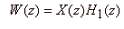or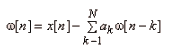and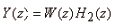or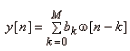The realization is shown in figure 9.8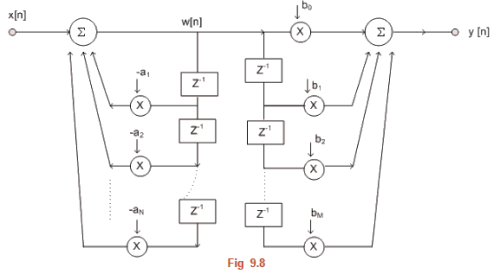Upon close examination of Fig 9.8, it can be seen that the two branches of delay elements can be combined as they both refer to delayed versions of ω[n] and upon simplification, the direct form II canonical realization is obtained as shown in figure 9.9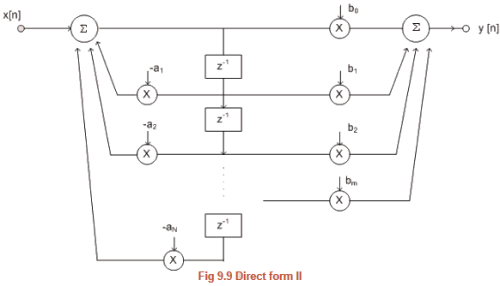In this form the number of delay element is max (M,N). It can be shown that this is the minimum number of delay elements that are required to implement the digital filter. This does not mean that this is the best realization. Immunity to roundoff and quantization are very important considerations.

An important special case that is used as building block occurs when. Thus H(z)  is ratio of two qualities in z-1, called biquadratic section, and is given by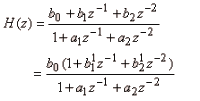The alternative form is found to be useful for amplitude scaling for improving performance file filter operation. This form is shown in figure 9.10.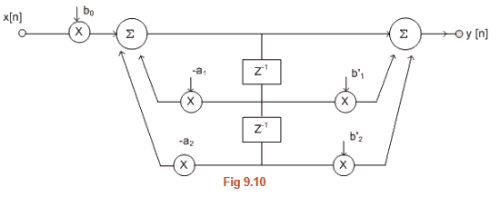Cascade RealizationsIn the cascade realization H(z) is broken into productof transfer functions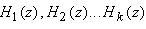each a rational expression in z-1 as follows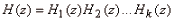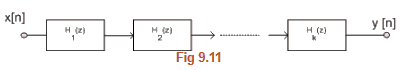H(z)  could be broken up in many ways; however the most common method is to use biquadratic sections. Thus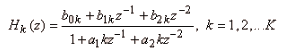by letting b2k and a2k  equal to zero we get bilinear section. Even among the biquadratic sections we have many choices as how we pair poles and zeros. Also the order of the sections can be different

Example: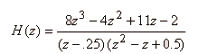Using only real coefficients H(z)  can be decompressed as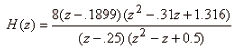Divides both numerator and denominator by z3 and factoring 8 as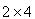, one possible rearrangement for H(z) is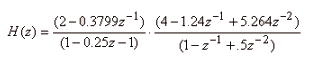This can be realized as shown is figure 9.12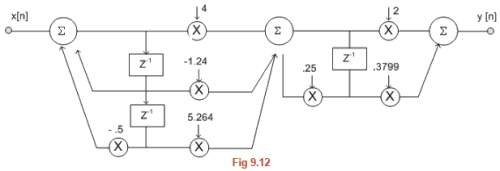Parallel Realizations:

The transfer function (z) could be written as a sum of transfer functions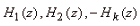as follows: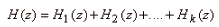One parallel form results when Hk(z) are all selected to be of the following form for (M < N)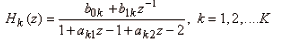If M > N, we will have a section H0(z) of FIR filter, obtained by performing long division. Once denominator polynomial has degree more than the numerator polynomial we perform the partial fraction expansion. The resulting structure is shown in figure 9.13.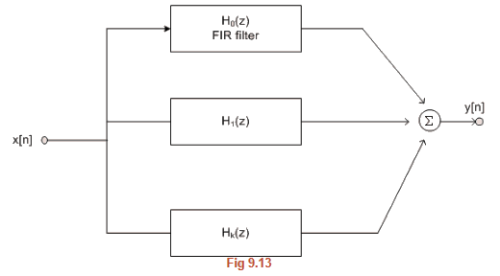Example:

Find the parallel form for the filter given in last example.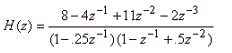Using MATLAB program or otherwise we get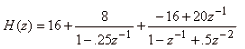using direst form realization for individual section we get the structure shown in figure 9.14.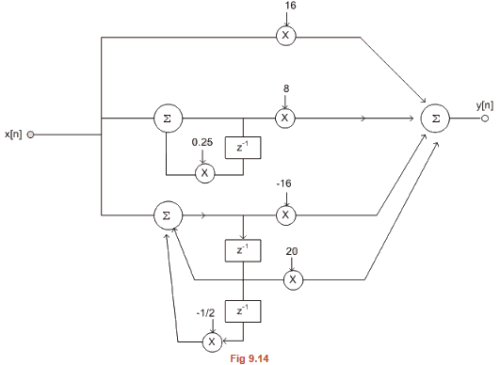Apart from these there exist a number of other realizations like lattice form, state variable realization etc.

The document FIR Filter Design & Realizations of Digital Filters Notes | Study Signals and Systems - Electrical Engineering (EE) is a part of the Electrical Engineering (EE) Course Signals and Systems.
All you need of Electrical Engineering (EE) at this link: Electrical Engineering (EE)Use Code STAYHOME200 and get INR 200 additional OFF Use Coupon Code

Top Courses for Electrical Engineering (EE)Signals and Systems

32 videos|74 docs|56 tests

Top Courses for Electrical Engineering (EE)Track your progress, build streaks, highlight & save important lessons and more!

,

,

,

,

,

,

,

,

,

,

,

,

,

,

,

,

,

,

,

,

,

;